Skip to main content

# Design of a passive, iso-elastic upper limb exoskeleton for gravity compensation

## Abstract

An additional mechanical mechanism for a passive parallelogram-based exoskeleton arm-support is presented. It consists of several levers and joints and an attached extension coil spring. The additional mechanism has two favourable features. On the one hand it exhibits an almost iso-elastic behaviour whereby the lifting force of the mechanism is constant for a wide working range. Secondly, the value of the supporting force can be varied by a simple linear movement of a supporting joint. Furthermore a standard tension spring can be used to gain the desired behavior. The additional mechanism is a 4-link mechanism affixed to one end of the spring within the parallelogram arm-support. It has several geometrical parameters which influence the overall behaviour. A standard optimisation routine with constraints on the parameters is used to find an optimal set of geometrical parameters. Based on the optimized geometrical parameters a prototype was constructed and tested. It is a lightweight wearable system, with a weight of 1.9 kg. Detailed experiments reveal a difference between measured and calculated forces. These variations can be explained by a 60 % higher pre load force of the tension spring and a geometrical offset in the construction.

## Background

Passive gravity compensation systems have existed for a long time  and many applications have evolved over the last century. One of the most well known applications is the parallel beam and spring systems used to balance lamp shades . These systems were adapted to balance TV screens  and to reduce holding and actuation torques in robot arms .

In recent years, exoskeletons have gained significant attention in the research and development community  mainly for medical rehabilitation , medical assistance  and military applications . Most exoskeleton developers focus on using electrically driven motors to support arm movement, ambulation or to carry objects on the back. Manipulation of heavy goods as seen in industrial applications are however rarely addressed. The main obstacles in these applications are the restricted power availability and the weight and volume of the exoskeleton.

Spring based systems have the advantage that no electrical power is needed, which reduces the weight and volume of the exoskeleton. Similar passive concepts do exist. However, due to the additional load, systems with counterweights are too heavy. In addition, springs with zero free length (i.e. ideal springs) compared to non-zero free length springs are costly or require an increased complexity . For simplicity reasons, the solution focuses on using non-zero free length springs.

### Requirementes

The design of this passive gravity compensation system targets the use as an upper limb (arm) exoskeleton. This arm exoskeleton should support the lifting of objects up to 7.5 kg. For this passive exoskeleton, no external power source is wanted, i.e. no sensors or powered actuators can be used. The gravity compensating force needs to be generated by spring forces only. Other main system characteristics are a minimal weight and a wearable, robust and compact design. The following requirements should be met:

1. 1.

minimum supporting force: 40 N

2. 2.

maximum supporting force: 120 N (these correspond to 4.5 kg of users arm weight plus 7.5 kg of external load)

3. 3.

adaptable to all supporting forces with a simple mechanism

4. 4.

close to constant gravity compensating force over a wide operating range (i.e. iso-elasticity) for all supporting forces

5. 5.

the vertical range of the compensation should cover at least $$\pm 45 {^{\circ }}$$ from a horizontal position

6. 6.

feasible and manufacturable geometric dimensions

7. 7.

minimal friction and lightweight design (≤4.5 kg) to minimize the energy required to carry, move and accelerate the exoskeleton

8. 8.

based on commercially available springs.

### Basic parallelogram—iso-elasticity

The early lamp poising systems consist of a parallelogram structure with a spring, Fig. 1. The parallelogram shown in Fig. 1—called the standard parallelogram—is actuated by an extension coil spring fixed diagonally within the parallelogram between points A and B. An external load acts on the right bar in the z-direction denoted by force $$F_z$$. Using vector addition, the resulting tension and compression forces in the bars $$a,\,b$$ and c are related to their geometric lengths, i.e.

\begin{aligned} \frac{F_a}{a}=\frac{F_b}{b}=\frac{F_c}{c}. \end{aligned}
(1)

In equilibrium, the force $$F_b$$ corresponds to the external load force, hence

\begin{aligned} F_b&=-F_z \end{aligned}
(2)

and the force in $$F_c$$ corresponds to the restoring force generated by the spring. Assuming that the spring is an ideal spring, the force is

\begin{aligned} F_c&=F_{\text {spring}}=k\,c, \end{aligned}
(3)

where k is the spring constant. Under this assumption, the lifting force $$F_b$$ is

\begin{aligned} F_b = \frac{b}{c}\,F_\text {spring} = \frac{b}{c}\,k\,c = k\,b = \text {const}. \end{aligned}
(4)

For an ideal spring, the lifting force $$F_b$$ is therefore independent of the spring length c and hence the lifting force is constant for any value of $$\varphi$$. This means that no external force or torque is required to balance the weight in any orientation of the mechanism within its workspace for a predefined load force $$F_z$$. This characteristic is often referred to as “iso-elasticity” .Fig. 1

A second feature of this parallelogram spring system is that dynamic movements from one side cause no movements on the other of the parallelogram as Eq. (4) is independent of $$\varphi$$ and if frictionless joints are assumed. This feature is utilised in stabilisation applications for motion picture cameras . It is also found in support systems for workers for lifting tasks and as a balancer and stabiliser for heavy tools .

### Effects of non-ideal springs and friction

In reality it is difficult to produce close-to-ideal springs. Figure 2 shows a selection of springs produced by Durovis AG, Switzerland (http://www.durovis.ch) compared to the ideal spring (zero free length) characteristics. Since a real spring always has a fixed free length $$l_0$$, a tension spring with a high pretension $$F_0$$ is needed to reproduce the ideal spring characteristic indicated by the solid line with endpoints in Fig. 2. Therefore, the restoring force needs to be described as

\begin{aligned} F'_{\text {spring}}=k\,\left( c-l_0\right) +F_0. \end{aligned}
(5)

Preload values are typically only 3–8 % of the desired values as shown in Fig. 2. These realistic values for the spring parameters lead to a strong non-constant behaviour of the supporting force of the mechanism with forces that are too low at upper- and too high at lower positions. Again the lifting force $$F_b$$ needs to be

\begin{aligned} F_b = \frac{b}{c}\,F'_\text {spring}&= \frac{b}{c}\left[ \,k\,\left( c-l_0\right) +F_0\right] \end{aligned}
(6)
\begin{aligned}&= \frac{b}{c}\left( F_0 -k\,l_0\right) +k\,b, \end{aligned}
(7)

where the variable length c can be substituted with the geometrial condition

\begin{aligned} c&= \sqrt{a^2 + b^2 - 2\,ab\,\sin (\varphi )}. \end{aligned}
(8)

Hence, for a realistic spring, the system will always equilibrate at a specific angle $$\varphi$$ for a given load $$F_z$$ (see Fig. 3, solid line marked b). Obviously, there is no iso-elastic behaviour. The original lamp poising systems still worked nicely because the joints were not frictionless. For the motion picture camera stabilization however, the friction in the joints is unwanted because it weakens the effect of decoupling the motion between the person and the carried camera attached to different sides of the parallelogram structure.Fig. 2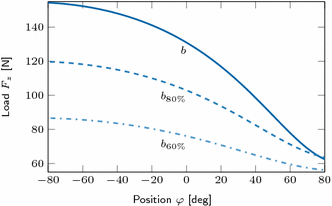Fig. 3

### Adaptability to load changes

For the ideal spring case, the parallelogram can be used to support different loads $$F_z$$ and exhibit iso-elastic behaviour. This can be achieved by changing the length b in Eq. (4). The same approach can be used for a system with a real spring. In Fig. 3, the equilibrating position $$\varphi$$ is shown versus the corresponding load $$F_z$$ for bar length b, and for bar lengths $$b_{80 \, \%}$$ and $$b_{60 \, \%}$$, which are 80 and 60 % of length b, respectively.

For shorter bar lengths, the difference between maximal and minimal force reduces. Hence, increasing the desired load compensation means that the system moves further away from an iso-elastic behaviour and therefore further away from the desired behaviour.

Two main options exist to manipulate Eqs. (7) and (8) to meet iso-elasticity and adaptability requirements. These are adjustable spring constants or a novel geometry, respectively. In , variable stiffness springs are used, which allows adjustment of the point of equilibration. The design is based on a nut with pitch equal to the spring. By changing the location of the nut along the length of the spring, sections of the spring are inactivated. This is a viable solution if the adjustment can be made while the spring is not elongated. For variable loaded springs (i.e. variable elongated springs), the pitch changes and therefore the adjustment of the stiffness is not easily possible.

In the next section, a geometrical change is proposed and optimised, which addresses the above-mentioned requirements.

## Extension by a 4-link mechanism

If we consider the behaviour of the standard parallelogram with realistic values for the spring, the spring forces are typically too low for small extensions and too high for large extensions of the spring. The basic idea for the additional mechanism is to move point A in Fig. 1 (fixture of the spring) in a way that the spring is tensioned more in upper positions and less in lower positions relative to the standard parallelogram. This is achieved by the additional 4-link mechanism shown in Fig. 4. It depicts the mechanism in the two extreme positions, upper and lower. The additional bars are denoted y, z and r. The lengths x, y and z are constant whereas the length of bar r can be adjusted along the axis $$\overline{OZ}$$. The spring is connected at point Y and it can be seen that the quadrilateral spanned by points OXYZ “flattens” out when moving from the upper to the lower position, which reduces the effective distance between points Y and B compared to the case when point Y would stay at the same location. In addition, the reduced distance between O and Y has the same effect as reducing length b in the standard parallelogram from Fig. 1.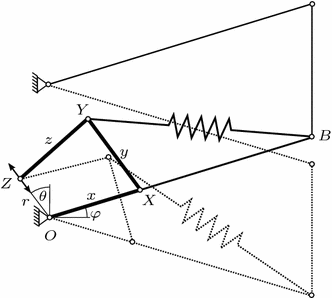Fig. 4

Angles and lengths are defined according to Fig. 5. The load force $$F_z$$ acts on the end effector at point B. By taking the sum of moments around the origin O, as shown in Fig. 6, the resultant force $$F_{z}$$ is as follows:

\begin{aligned}&F_{z}\,a\,\cos \left( \varphi \right) +F_y\,x\,\sin \left( \alpha \right) -F_{c}\,a\,\sin \left( \beta \right) = 0\end{aligned}
(9)
\begin{aligned}&F_{z} = \frac{F_{c}\,a\,\sin \left( \beta \right) -F_y\,x\,\sin \left( \alpha \right) }{a\,\cos \left( \varphi \right) } \end{aligned}
(10)

where $$F_c$$ and $$F_y$$ are the spring force and the force in bar y, respectively. Using the geometrical arguments illustrated by Fig. 5, the force $$F_y$$ is:

\begin{aligned} F_{y}=\frac{F_{c}\,\sin \left( \gamma +\alpha -\beta \right) }{\sin \left( \gamma \right) }. \end{aligned}
(11)

By substituting $$F_y$$ by Eq. (11) in Eq. (10), the final resultant force as a function of spring force $$F_c$$ and internal angles is:

\begin{aligned} F_{z}=F_{c}\left[ \frac{\sin \left( \beta \right) }{\cos \left( \varphi \right) }-\frac{x\,\sin \left( \gamma +\alpha -\beta \right) \sin \left( \alpha \right) }{a\,\sin \left( \gamma \right) \cos \left( \varphi \right) }\right] . \end{aligned}
(12)

Hence, the force $$F_z$$ can be described in terms of the spring force $$F_c$$—a real spring characteristic is assumed according to Eq. (7)—and geometrical relations which depend on the lengths of the four bars x, y, z and r. In Eq. (12) the angles $$\alpha$$, $$\beta$$ and $$\gamma$$ can be algebraically replaced by the bar lengths. The expression is however omitted here for the sake of compactness.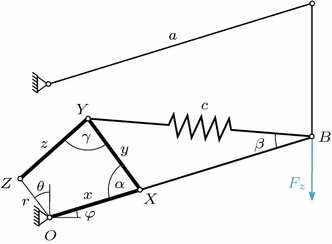Fig. 5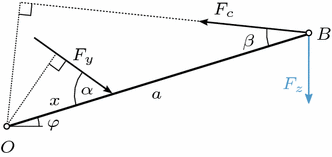Fig. 6

## Optimisation of the geometry

Qualitatively, the 4-link mechanism provides the desired behaviour. However, the design question remains as to how the dimensions of three bar lengths x, y and z (according to Fig. 5) need be chosen to provide a reasonable iso-elastic behaviour. For this purpose, a design optimisation problem is formulated. For practical reasons, it was decided to move point Z on a straight line starting at origin O to vary the supporting force $$F_z$$. The pitch angle $$\theta$$ is introduced as an additional parameter to be optimised. The length r should vary from 5 mm (40 N support) to approximately 45 mm (120 N support). The working range of the parallelogram was set to a range of $$\varphi = -45^\circ \ldots +45^\circ.$$

To reach an iso-elastic behaviour over the working range, the curves in Fig. 3 need to collapse to horizontal lines. A simple optimisation criteria is therefore to use the difference between the maximally and minimally generated forces $$F_z$$ within the operating range. Therefore, the optimisation reads as follows

\begin{aligned} \underset{x,y,z,\theta }{\min } R(x,y,z,\theta |r,\varphi ) \end{aligned}
(13)

with

\begin{aligned} R(x,y,z,\theta |r,\varphi ) =\max (F_z(\varphi |r))-\min (F_z(\varphi |r)). \end{aligned}

The notation $$F_z(\varphi |r)$$ means: the function $$F_z(\varphi )$$ at a given value r. In the present form an optimisation would favour geometrical parameters that lead to small absolute values for the lifting force. Thus an extra penalty term is added to the objective function R which measures the maximum distance of forces at r = 45 mm to the desired maximum force of 120 N.

In principle the three parameters of the spring (spring constant, length and pretension) could also be values to be optimised. But since the manufacturing process strongly restricts these values, it was decided to select a spring and calculate the values above for this particular spring. The values of the spring were chosen from energetic considerations like:

\begin{aligned} (l_\text {max}-l_0)\cdot \frac{F_\text {max}-F_0}{2}>m\,g\,h, \end{aligned}
(14)

which means that the total energy in the maximum tensioned spring must be greater than the total lifting energy of the mass.

For manufacturing reasons the geometrical parameters x, y, z and $$\theta$$ are constrained to reasonable values. Their limits were given as

\begin{aligned} x,y,z&\in [40 \, \text {mm},\,100 \, \text {mm}],\\ \theta&\in [10^\circ ,\,80^\circ ]. \end{aligned}

The resulting force $$F(\varphi ,r)$$ can be calculated according to Eq. (12).

The optimisation is done using Matlab (The MathWorks, Inc.) and the fmincon function of the optimisation toolbox. The used algorithm uses interior-point approach. The function $$F(\varphi ,r)$$ was computed on a discrete grid of $$\varphi$$ and r values.

The optimised geometrical parameters are $$x=58.2 \, \text{mm}$$, y = 72.9 mm, z = 94.3 mm and $$\theta =51.4^{\circ }$$. Note that all values lie inside the given bounds. Figure 7 shows the resulting force values at the end effector for different angles $$\varphi$$ and different settings of the point Z by varying distance r from 5 ... 45 mm. For small lifting forces (small values of r) nearly perfect iso-elastic behaviour can be reached. The maximum force at 120 N shows a variation of ±3.2 N for positions $$\varphi = -45^{\circ } \ldots 45^{\circ }.$$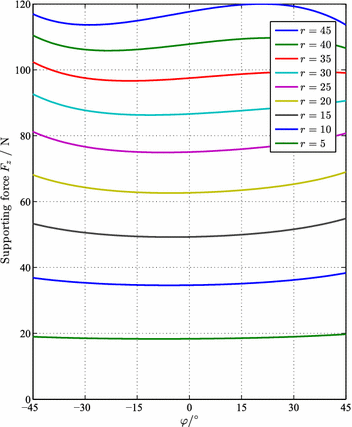Fig. 7

If we consider the optimisation with respect to the four parameters $$x,\,y,\,z,\,\theta$$ one has to ask whether these four parameters give a proper description of the optimisation problem. Figure 8 shows a stacked plot of the optimisation function $$R(x,y,z,\theta |r,\varphi )$$ under variation of the parameters $$x,\,y,\,z$$. The angle $$\theta$$ is kept at the optimised value of $$51.4^\circ$$. For illustration purposes the z dimension is quantised. The figure shows only a very slight variation of R if we move diagonally through the parameter space shown by the dark blue areas on each surface plot. Note that the objective function has very large values at outer regions of the shown parameter space. For this reason the color bar has a nonlinear scale at the outer end. The optimisation routine has to find an optimum in this “flat valley”, but there does seem to be a global optimum. However, there are many other possible combinations of the geometrical parameters with similar iso-elastic behaviour to that of the optimised parameters shown above.Fig. 8

## First prototype

Figure 9 shows the prototype based on the 4-link mechanism. In the lower left, indicated by R, the adjustment for moving the supporting point Z can be seen. It is a nut running along a spindle when lever L is turned. By that, the desired lifting force can be adjusted.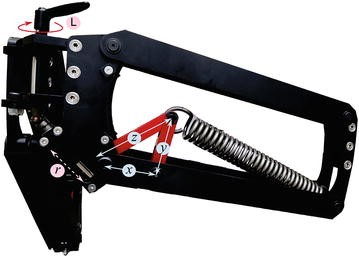Fig. 9

The total weight of one parallelogram is 1.9 kg. As in other applications, two parallelogram segments are connected in series to provide a reasonable range of operation. The forearm of the user is attached to the end of the second segment using a cuff. This specific design supports up to 120 N at the location of the cuff. Considering that an arm consists of approximately 5 % of the human bodyweight, this system can therefore support an external load up to the required 7.5 kg. This is at the upper limit for most people when the load is held in the hand. A tool mounted directly to the system could weigh up to 12 kg. Ball bearings were used to reduce friction in the joints.

### Experimental verification

Tests were carried out to verify the behavior of the developed system. Using different weights and a spring balance, the effective lifting force at 7 different angles $$\varphi$$ were measured. The weights correspond to 20, 50, 70, 90 N and since there is a static friction of approximately ±5 N, the measurements were done in an upward and a downward direction. The force was applied with a hand held spring scale. A weight of 7 N was added which is the contribution to the weight of the arm itself. Figure 10 on the left shows results of the theoretical curve and the measurements. For each weight and angle the two squares show the upward and downward direction of the measurement. Especially for higher loads there is a significant discrepancy between calculated and measured forces. The supporting force tends to higher values at larger angles $$\varphi.$$Fig. 10

The reason for this discrepancy was found in a considerably difference of the spring data and also a geometrical effect which comes from the attachment of the spring to the construction (a small offset of a bolt). The spring characteristics was validated on a tensile testing machine. The values of the spring characteristics in the optimisation was taken from the datasheet an was: length $$l_0=178.75$$ mm, preload force: $$F_0=115$$ N, spring rate: $$c=11.15$$ N/mm. The measured values were found as: length $$l_0=178.75$$ mm, preload force: $$F_0=191.9$$ N, spring rate: $$c=10.9$$ N/mm.

Figure 10 on the right shows the measurements and calculated values with these corrected spring parameters. These values will be used in future for a new optimization for a second prototype. The first prototype was assembled to a supporting system (2 × 2 parallelograms for two arms) and show very positive results in tests (see Fig. 11).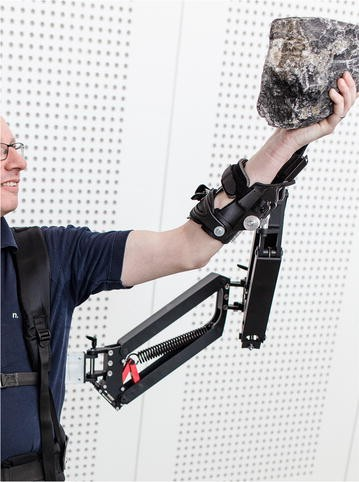Fig. 11

## Conclusion and outlook

The resulting design exhibits close to iso-elastic behaviour over a wide operating range and is adjustable for loads in the range of 40–120 N. This makes the mechanism attractive for a lightweight exoskeleton arm, which is fully passive but still sufficiently powerful to support significant weight. The same mechanism can be used to balance an object such as a tool. Compared to electrically powered exoskeleton arms, the supporting force is continuously present and cannot be switched on or off depending on whether an object is being carried or not. The design is ideal for supporting the user’s posture and for lower load weights.

The design optimisation approach provided an efficient framework for selecting the best parameters. Future development priority lies in further reducing the weight and designing the exoskeleton arm to be more suitable for specific tasks.

## References

1. 1.

Lu Q, Ortega C, Ma O (2011) Passive gravity compensation mechanisms: technologies and applications. Recent Patent Eng 5:32–44

2. 2.

Carwardine G (1932) Improvements of elastic force mechanisms. UK Patent Specification 379,680

3. 3.

Jacobsen J (1962) Adjusting means for a lamp structure. US Patent 3,041,060

4. 4.

French MJ, Widden MB (2000) The spring-and-lever balancing mechanism, george carwardine and the anglepoise lamp. Proc Inst Mech Eng, Part C 214:501–508

5. 5.

Leporati RA (1968) Counterpoising or equipoising mechanism. US Patent 3,409,261

6. 6.

Chalfoun J, Bidard C, Keller D, Perrot Y, Piolain G (2007) Design and flexible modeling of a long reach articulated carrier for inspection. In: IEEE/RSJ international conference on intelligent robots and systems, pp 4013–4019

7. 7.

Agrawal A, Agrawal SK (2005) Design of gravity balancing leg orthosis using non-zero free length springs. Mech Mach Theory 40(6):693–709. doi:10.1016/j.mechmachtheory.2004.11.002 Accessed 26 Jan 2016

8. 8.

Brown GW (1979) Equipment support system. US Patent 4,156,512

9. 9.

Goldman D, Kelly H, Pagliery J (2014) 36 Coolest gadgets of 2014. CNNMoney. http://money.cnn.com/gallery/technology/innovationnation /2014/12/10/coolest-gadgets-2014/24.html

10. 10.

Pons JL (ed) (2008) Wearable robots: biomechatronic exoskeletons. Wiley, Hoboken

11. 11.

Rosen J, Brand M, Fuchs MB, Arcan M (2001) A myosignal-based powered exoskeleton system. IEEE Trans Syst Man Cybernet Part A Syst Humans 31(3):210–222

12. 12.

Nef T, Mihelj M, Kiefer G, Perndl C, Muller R, Riener R (2007) ARMin-exoskeleton for arm therapy in stroke patients. In: Proceedings of the 10th international conference on rehabilitation robotics (ICORR), pp 68–74

13. 13.

Banala SK, Kim SH, Agrawal SK, Scholz JP (2009) Robot assisted gait training with active leg exoskeleton (ALEX). IEEE Trans Neural Syst Rehabil Eng 17(1):2–8

14. 14.

Kong K, Jeon D (2006) Design and control of an exoskeleton for the elderly and patients. IEEE/ASME Trans Mech 11(4):428–432

15. 15.

Kazerooni H, Steger R (2006) The berkeley lower extremity exoskeleton. Trans ASME 128:14–25

16. 16.

Dorsser WDV, Barents R, Wisse BM, Schenk M, Herder JL (2008) Energy-free adjustment of gravity equilibrators by adjusting the spring stiffness. Proc Inst Mech Eng Part C 222(9):1839–1846

17. 17.

Byrd RH, Gilbert JC, Nocedal J (2000) A trust region method based on interior point techniques for nonlinear programming. Math Program 89(1):149–185

Download references

## Authors’ contributions

RA proposed the extended gravity compensation mechanism and supervised the prototype design and contributed to the parameter optimisation. DS developed the prototype and contributed to the parameter optimisation. KSS contributed to the background information. All authors contributed equally to paper preparation and revision. All authors read and approved the final manuscript.

### Acknowledgements

This mechanism was developed within the project Robo-Mate. The Robo-Mate project has received funding from the European Union’s Seventh Framework Programme for research, technological development and demonstration under Grant Agreement No. 608979.

### Competing interests

The authors declare that they have no competing interests.

## Author information

Authors

### Corresponding author

Correspondence to Ruprecht Altenburger.

## Rights and permissions

Open Access This article is distributed under the terms of the Creative Commons Attribution 4.0 International License (http://creativecommons.org/licenses/by/4.0/), which permits unrestricted use, distribution, and reproduction in any medium, provided you give appropriate credit to the original author(s) and the source, provide a link to the Creative Commons license, and indicate if changes were made.

Reprints and Permissions